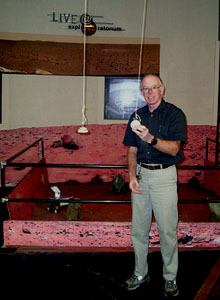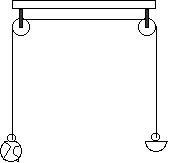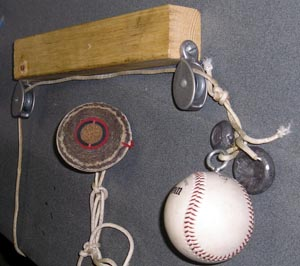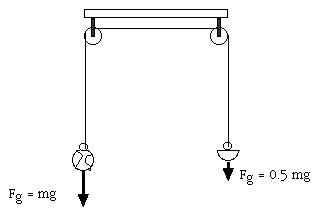Mars Acceleration of Gravity

What's an Atwoods machine?A baseball counterweighted by a half baseball accelerates with Martian acceleration.

Introduction

An Atwoods machine can be used to create the acceleration of gravity found on Mars here on earth. An Atwoods machine uses pulleys and a counterweight to slow down the acceleration of gravity.

Material

An object such as a baseball.

A second baseball to drop under earth gravity.

A counterweight object that has a mass equal to half the mass of the baseball, such as a baseball cut in half on a bandsaw.

Two pulleys

Cord, several meters (yards) long, slightly longer than the distance from the ceiling to the floor.

Screw eyes to attach the cord to the baseballs.

A wood two-by-four to hold the pulleys apart, 2/3 meter (2 feet) long.

A way to attach the two-by-four to the ceiling.

AssemblyDeatils of the Mars baseball atwoods machine.

Attach the screw eyes to the baseball and the half-baseball.

Attach the pulleys to the ends of the two-by-four.

Run the cord through the pulleys and tie the ends of the cord to the baseballs.

Mount the two-by-four and pulleys to the ceiling.

To Do and Notice

Lift up the baseball from the floor.

Drop it.

Notice how it accelerates toward the ground.

Drop a free baseball and notice its acceleration.

The acceleration of the free baseball on earth three times greater than the acceleration of the baseball under Martian conditions.

Gently toss the baseball upward.

Notice how it easily goes skyward, then slowly accelerates back down to earth.

Repeat the toss with the free baseball.

What's Going On?

Gravity pulls down on the baseball and the half-baseball.

The tension in the string between the baseball and the half-baseball pulls up on the whole baseball countering the force of gravity.

Thus the baseball accelerates toward the ground more slowly.

Math Root

When the whole baseball accelerates toward the ground the half-baseball must accelerate at exactly the same rate since they are connected by a cord.Gravity forces on the baseball and half-baseball.

Thus using Newton's famous third Law,

F = Ma

where F is the total force on the system of two connected baseballs, M is the mass of both baseballs, and a is their acceleration, we find

a = F/m

Now the force on the whole baseball due to gravity is mg

where g is the acceleration of gravity on earth.

The force of gravity on the other half-baseball is in the opposite direction and is -0.5 mg. The negative sign indicates the opposite direction.

So the total, or net, force is 0.5 mg. A downward force equal to half the weight of the baseball.

Yet both the baseball, of mass m, and the half baseball, of mass 0.5 m, must accelerate so the mass of the two together is M = m + 0.5 m = 1.5 m.

The resulting acceleration is

a = F/M = 0.5 mg/ 1.5m = 0.33g one third the acceleration of gravity on earth.

Due to the mass of the cord and also the inertia of the pulleys you may have to add some lead weights to the whole baseball to adjust the system so that it accelerates at ars gravity. I had to add six ounces of lead fishing weights to my whole baseball to make the system work quantitatively.

Going Further

The accelration of an Atwoods machine on earth in g's when a mass of 1 is balanced against a mass of x is

a = (1-x)/(1+x)

We can use this to calculate the fraction, x, of a full baseball needed to counterweight a baseball to give the surface acceleration of gravity on other worlds.

Try this for Titan, a moon of Saturn where the surface acceleration is 0.13 g or about 1/8 g

Try it for the moon where the acceleration of gravity is 1/6 g.